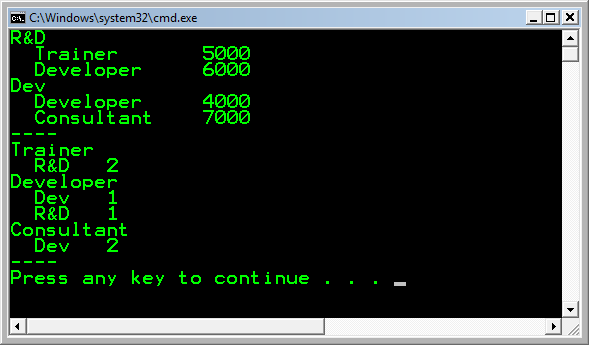# C# LINQ Pivot() Function

22-jan-2009

I have created a LINQ extension method which can be used to Pivot an enumeration. Just like you can PIVOT a SQL Query in SQL Server.

The following example uses the Pivot() function to group Employees by Department and Function and aggregates the Salary using a SUM. The second example uses a different grouping and Counts the number of employees per group.

```class Program {

internal class Employee {
public string Name { get; set; }
public string Department { get; set; }
public string Function { get; set; }
public decimal Salary { get; set; }
}

static void Main(string[] args) {

var l = new List<Employee>() {
new Employee() { Name = "Fons", Department = "R&D",
Function = "Trainer", Salary = 2000 },
new Employee() { Name = "Jim", Department = "R&D",
Function = "Trainer", Salary = 3000 },
new Employee() { Name = "Ellen", Department = "Dev",
Function = "Developer", Salary = 4000 },
new Employee() { Name = "Mike", Department = "Dev",
Function = "Consultant", Salary = 5000 },
new Employee() { Name = "Jack", Department = "R&D",
Function = "Developer", Salary = 6000 },
new Employee() { Name = "Demy", Department = "Dev",
Function = "Consultant", Salary = 2000 }};

var result1 = l.Pivot(emp => emp.Department,
emp2 => emp2.Function, lst => lst.Sum(emp => emp.Salary));

foreach (var row in result1) {
Console.WriteLine(row.Key);
foreach (var column in row.Value) {
Console.WriteLine("  " + column.Key +
"\t" + column.Value);

}
}

Console.WriteLine("----");

var result2 = l.Pivot(emp => emp.Function,
emp2 => emp2.Department, lst => lst.Count());

foreach (var row in result2) {
Console.WriteLine(row.Key);
foreach (var column in row.Value) {
Console.WriteLine("  " + column.Key +
"\t" + column.Value);

}
}
Console.WriteLine("----");
}
}
```

The results in:Tags: CSharp

Name
Comment
5 + 7 =

• peter
17 jul 2019 10:41